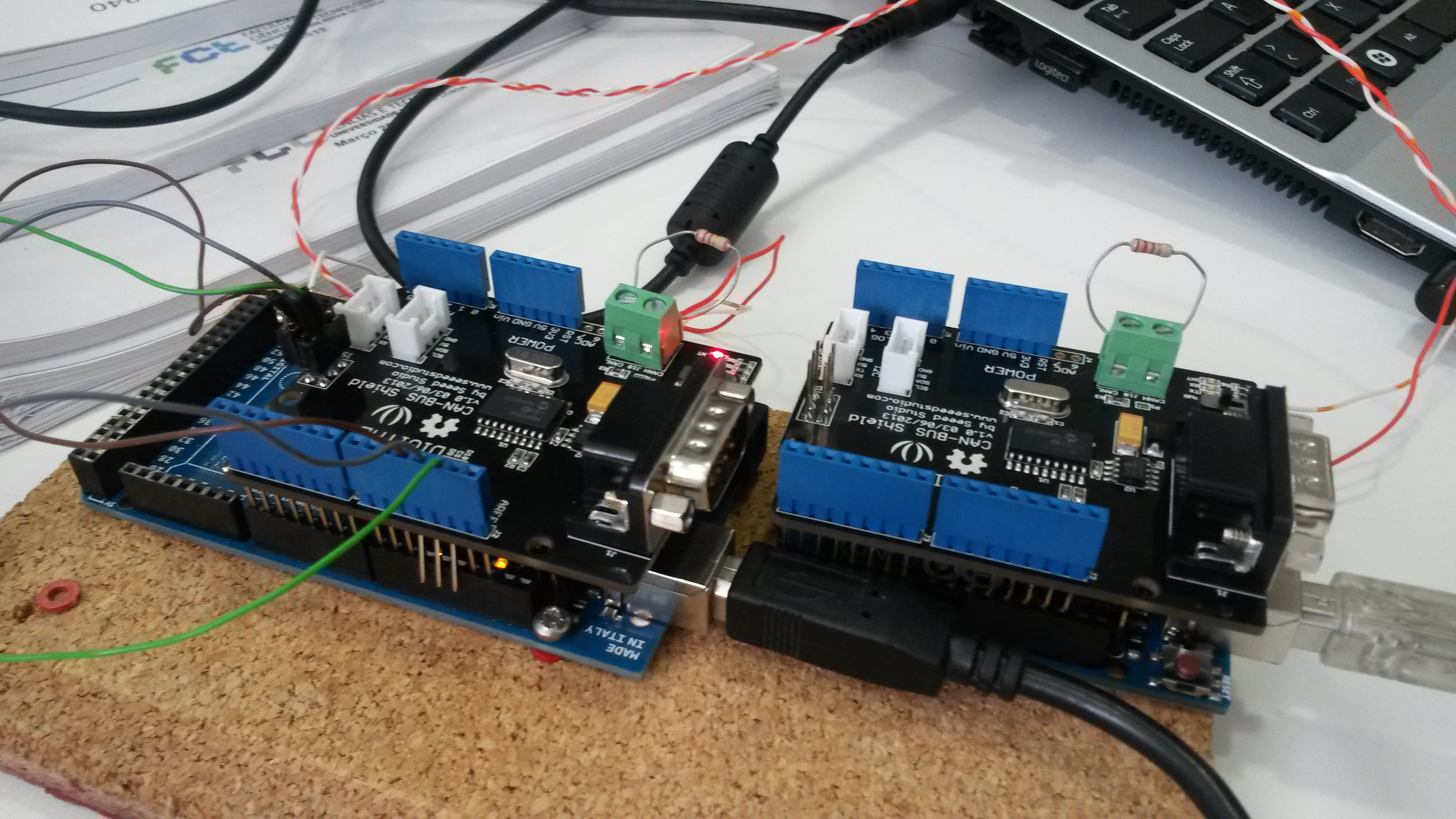# CAN Bus-Shield sending data

Hello everyone, I’m studying the CAN protocol and plan to implement it with CAN bus shield. My question is if I can send data in float or double format.Actually it’s not question of CAN BUS Shield, just like if you can send a float via I2C?

yes, it can send any data format, but it need some interface.

such as:

// send
send_float_can(float dta);

make_float_can(unsigned char *dta);

I had wrote a test code, just have a try:

[code]void make_float_to_array(float f, unsigned char dta)
{
memcpy(dta, (unsigned char
)&f, sizeof(float));
}

void make_array_to_float(float *f, unsigned char dta)
{
memcpy((unsigned char
)f, dta, sizeof(float));
}

void setup()
{
Serial.begin(115200);

``````float a = 5.12;

unsigned char array;

make_float_to_array(a, array);

for(int i=0; i<4; i++)
{
Serial.print("0x");
Serial.print(array[i], HEX);
Serial.print('\t');
}

Serial.println();

float b = 0;

make_array_to_float(&b, array);

Serial.print("b = ");
Serial.println(b);
``````

}

void loop()
{

}[/code]

Thank you very much…